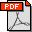Article Details

Surface MMR enhanced 3D inversion using model reconstruction strategy

Oleh   Yue Yang [-]
 Kontributor / Dosen Pembimbing : Aihua Weng, Sirui Li, Dajun Li, Shiwen Li, Jianping Li Jenis Koleksi : Jurnal elektronik Penerbit : Lain-lain Fakultas : Subjek : Kata Kunci : Magnetometric resistivity 3D inversion Non-linear conjugate gradient inversion Model reconstruction Sumber : Journal of Applied Geophysics 159 (2018) 193–203, https://doi.org/10.1016/j.jappgeo.2018.08.015 Staf Input/Edit : File : 1 file Tanggal Input : 2019-01-10 15:11:042018_EJRNL_PP_YUE_YANG_1.pdf

Terbatas
» Gedung UPT Perpustakaan

Previous inversions of surface magnetometric resistivity (MMR) could obtain only the ratio, not the absolute value of earth electrical conductivity. In this paper, instead of solving Poisson's equation, the magnetic fields generated by low frequency alternating current(AC) line are looked as MMR data and calculated from electric- type Helmholtz's equation in frequency domain, so that the absolute conductivity could be taken into account. Forward modeling scheme is adapted from staggered-grid finite-difference method. Inversion algorithm is non-linear conjugate gradient (NLCG). The magnetic field perpendicular to line source is selected as observed data. To improve depth resolution, we introduce a model reconstruction strategy based on reference model. In the strategy, the upper portion of the resultant model from previous inversion is replaced by a homogeneous layer to construct a new model to trigger next inversion. The thickness of the homogeneous layer is called reconstruction depth, it is equal to the depth where the models difference between last two iterations after the run of the ith inversion is almost none-zero. The reconstruction could be applied until the model difference is almost zero or the difference of model differences between two adjacent inversions is less than a predefined threshold. Inversion results from synthetic and real data have proved that the proposed multi-reconstruction inversion strategy is able to obtain absolute resistivity, as well as enhance the deep resolution of surface MMR data inversion

Pencarian Lanjut
Info Digilib ITB
Tautan